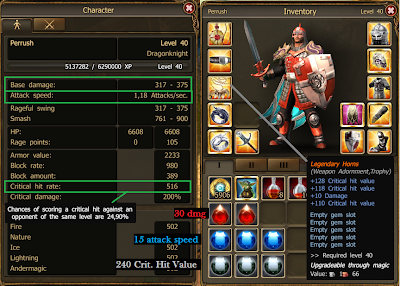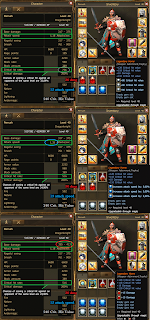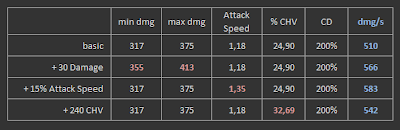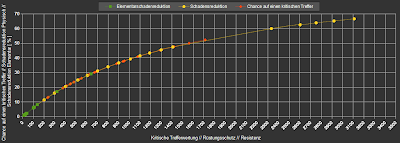## Monday, March 11, 2013

### 034 - Damage vs Attack Speed vs Critical Hit ValueThe 3 Offensive Gemstones (@ a 25% reduced price)

Question :

Which of the 3 offensive gemstones is the best to add ?

I hope to answer this question in this post.  Let me first say that a polished offensive gemstone normally costs 6400 Andermant and not 4800 like in the image above.  But today gemstones were on a 25% reduced price, that's why they are labelled as 4800 Andermant.

I will try to answer this question by adding 3 polished offensive gemstones (ruby, onyx and sapphire) at a time to my character and calculate total damage for each gemstone.

In that way we can see which gemstone has to most influence on total damage done.  But first let us make a formula to calculate that total damage done.

Formula for total damage per second doneCharacter values with no gemstones
In the image above you see my dragon knight's statistics.  The Legendary Horns have no gemstones inside, I call this my 'basic' character.   Mind that there are 3 (actually 4) values important to calculate damage done.

1) Base Damage :  Gemstone -> Ruby
This is the damage done with 1 stroke.  It has a minimum and maximum value.  So you damage done with a single stroke can vary between those 2 value.  For my calculation I use average damage which is the average of the minimum and maximum value.

avg dmg = (min dmg + max dmg ) / 2

2) Attack Speed :  Gemstone -> Sapphire
The attack speed value gives the number of strokes per second.  When we combine the avg dmg (which is the damage on average done by 1 stroke) with the attack speed (number of strokes per second) we get the damage per second.  In formula it looks like this :

avg dmg/s =  [(min dmg + max dmg ) / 2] * Attack Speed

3) Critical Hit Rate (CHR) : Gemstone -> Onyx

While damage and attack speed is pretty straightforward, CHR maybe needs some explanation.

To explain this we introduce the term 'Critical Hit'.
A critical hit is a hit where the normal damage is multiplied by the critical damage. The Chance of delivering a critical hit is given by the critical hit rate.
Now back to my basic character to explain this definition.  My CHR is 24.90% and the critical damage (CD) is 200%.  This means that in 24.90% a hits of mine does 200% of my avg damage.

This also means that in 100% - 24.90% = 75.1% I deliver a normal hit.  This 75.1% I refer to as Normal Hit Rate (NHR).

Calculating for total damage per second done :

part A : NHR

(100% - CHR)  *  [(min dmg + max dmg ) / 2] * Attack Speed

part B : CHR

CHR  *  [(min dmg + max dmg ) / 2] * Attack Speed * CD

Total formula = part A + part B
{(100% - CHR) * [(min dmg + max dmg ) / 2] * Attack Speed} + [CHR * ((min dmg + max dmg ) / 2) * Attack Speed * CD]

Explanation.  Part A gives the average dmg/s for normal hits.  Part B is the equation for a criticit hit.  Mind that the critical damage is added in this part.

Our Base Character

As we calculate the dmg/s for our base character we get :

- {(100% - 24.9%) * [(317 + 375) / 2] * 1.18} + [24.9% * ((317 + 375) / 2 * 1.18 * 200%]

- (75.1% * 346 dmg * 1.18 attack/s) + (24.9% * 692 dmg * 1.18 attack/s)

- (306.61 + 203.32) dmg/s

- 509.93 dmg/s

Although my character has only 375 maximum damage per swing, it does almost 510 dmg per second.  This is possible because it delivers more than 1 attack per second (1.18 to be precise) and because in 24.9% of the cases the damge done is dubble the normal damage.

Offensive gemstones compared

Now that we have our formula for calculation dmg/s, we can compare the effect of adding different gemstones.  As you can see in the image underneath, I added 3 polished gemstones (or a equivalent of that in case of the ruby) at a time.Values for each gemstone

In the table you can see the values for the different characters.Calculations for different gemstone additions

Adding 3 polished sapphire with a total of +15% attack speed seems to give you the most value for money.  It raised the dmg/s from 510 tot 583, that's a raise of 71 dmg/s or 14%.

Adding for 19200 Andermant 3 polished Onyx gemstones raises you dmg/s with 'only' 32 points !!  That's roughly half (read : -50%) of the effect of the sapphires.

The rubies or somewhat in between.  But there is still a 22% difference between the effect of the sapphires and those of the rubies.  Pretty significant imho.

Conclusion : Adding attack speed seems the best option if you want to inflict more damage.  Adding CHV seems to be the worst choice.

Some remarks

1) CHR increases not linear.  When you have an already high CHR, the effect of adding extra Onyx gemstones will be less than when you have a low CHR.  I found the image underneath on the German Forum in this post.CHR increase is not linear
2) Adding 30 dmg in gemstones increased my damage with 38 points instead of the expected 30.  This the effect of the base damage bonuses you get in the experience and knowledge tree.  The higher you are on those trees, the greater the effect will be.

3) Some items (mainly weapons) have enchantments which increases damage or attack speed percentage wise.  Adding gemstones of those enchantments will also increase the effect of that gemstone.

1.YOU HAVE DONE GREAT WORK WITH YOUR BLOG. VERY USEFULL FOR EVERYONE PLAYING DRAKENSANG. THANK YOU!

2."3) Some items (mainly weapons) have enchantments which increases damage or attack speed percentage wise. Adding gemstones of those enchantments will also increase the effect of that gemstone."

AFAIK, this only works with rubies in weapons that have % increased damage enchantments. I've tested the possibility of this with sapphires in a weapon that has % attack speed enchantments and it had no additional effect on the character's overall attack speed.

1.interesting, thanks for the info.

3.One comment against adding (too many) sapphires. The extra speed is good if you use your basic attack (and some skills without cooldown, for example chain lightning as a mage). But a meteor or destruction spell is not affected by sapphires, as they won't be faster, while a ruby increases the damage for all skills and spells. Therefore I usually vote for ruby.

1.it is best to use both rubies and attack speed gems. thanks for the info, i will try to balance both gems.

2.10 dmg stays 10 dmg wether you have 200 base dmg or 1500 base dmg. But adding sapphires increases your dmg per second according to your base dmg.

5% increase of 1000 dmg/s is 50 dmg/s. It takes a lot of rubys to get that dmg/s increase.

Just use the formula to see what's best ;)

3.4.kk, thanks for the info, i am a ranger, so adding speed is no problem. i don't use supportive shills like bird of prey often, mostly those skills the attack speed gems affect. i am adding 50% attack speed to my alans bow(+ 16% attack speed) and extra 30 + damage gems to my focus crystal.

5.Hey,
I`m ranger to, and i have legend bow with 1,43 speed in base (4 modificators of speed) and sapphires count with them. I have 2 sapphires (5% and 2,5%) and my end speed is 1,53 (i`m only 26lvl). But this modificator from bow is only working with sapphires (doesn't matter where you put it: 5% is in weapon decoration and 2,5% is in bow.

For example if you have +2.5% speed in other item (like wind arrow) then it will be only 2,5% added :)

I hope i helped.

6.thanks, that helped.

7.Hi nice work but what is gone wneh the Cd changes for example my is 275%

8.Im the person who post: AnonymousAugust 18, 2014 at 1:09 AM

This is easy to count. But it depends what is your base dmg. On lover base dmg is better to put rubys.

We can take 3 types of dmg (all will be avarage)

As - Your attack per second = 1

CD - Crit DMG = 275% (dosent matter how it is big it could be regular 200% because this value is constant for both ruby and sapphires)

What we gonna check:
DMG in one blow - HitDMG
Critt dmg in one blow - CHitDMG
Normal dmg per second- NDMG/s
Critt dmg per second - CDMG/s

HitDMG1 = ADMG1 = 100 = 100dmg
CHitDMG1 = ADMG1 * CD = 100 * 2,75 = 275dmg
NDMG/s 1 = ADMG1 *As = 100* 1 = 100dmg
CDMG/s 1 = ADMG1 * As *CD = 100 * 1 * 2,75 = 275dmg

After one RUBY (+10dmg)
HitDMG1 = (ADMG1 + 10) = 100 +10 = 110dmg
CHitDMG1 = (ADMG1 +10) * CD = (100+10)*2,75 = 302,5dmg
NDMG/s 1 = (ADMG1 + 10) * As = (100+10)*1=110dmg
CDMG/s 1 = (ADMG1 + 10) * As * CD = (100+10)*1*2,75 =302,5dmg

After one sapphire (5%) ("As" will be 1,05)
CHitDMG1 = ADMG1* CD = 100 * 2,75 = 275dmg
NDMG/s 1 = ADMG1 * As = 100*1,05= 105dmg
CDMG/s 1 = ADMG1 * As * CD = 100*1,05*2,75 = 288,75dmg

So for 100 Avarage DMG Ruby is better choice (only if you wanna check the DMG value)

HitDMG2 = ADMG2 = 200 = 200dmg
CHitDMG2 = ADMG2 * CD = 200 * 2,75 = 550dmg
NDMG/s 2 = ADMG2 *As = 200* 1 = 200dmg
CDMG/s 2 = ADMG2 * As *CD = 200 * 1 * 2,75 = 550dmg

After one RUBY (+10dmg)
HitDMG2 = (ADMG2 + 10) = 200 +10 = 210dmg
CHitDMG2 = (ADMG2 +10) * CD = (200+10)*2,75 = 577.5dmg
NDMG/s 2 = (ADMG2 + 10) * As = (200+10)*1=210dmg
CDMG/s 2 = (ADMG2 + 10) * As * CD = (200+10)*1*2,75 =577.5dmg

After one sapphire (5%) ("As" will be 1,05)
CHitDMG2 = ADMG2* CD = 200 * 2,75 = 550dmg
NDMG/s 2 = ADMG2 * As = 200*1,05= 210dmg
CDMG/s 2 = ADMG2 * As * CD = 200*1,05*2,75 = 577.5dmg

HitDMG3 = ADMG3 = 500 = 500dmg
CHitDMG3 = ADMG3 * CD = 500 * 2,75 = 1375dmg
NDMG/s 3 = ADMG3 *As = 500* 1 = 500dmg
CDMG/s 3 = ADMG3 * As *CD = 500 * 1 * 2,75 = 1375dmg

After one RUBY (+10dmg)
HitDMG3 = (ADMG3 + 10) = 500 +10 = 510dmg
CHitDMG3 = (ADMG3 +10) * CD = (500+10)*2,75 = 1402,5dmg
NDMG/s 3 = (ADMG3 + 10) * As = (500+10)*1=510dmg
CDMG/s 3 = (ADMG3 + 10) * As * CD = (500+10)*1*2,75 =1402,5dmg

After one sapphire (5%) ("As" will be 1,05)
CHitDMG3 = ADMG3* CD = 500 * 2,75 = 1375dmg
NDMG/s 3 = ADMG3 * As = 500*1,05= 525dmg
CDMG/s 3 = ADMG3 * As * CD = 100*1,05*2,75 = 1443,5dmg

Calculation was for 100%dmg skill but with skills with 125% (like precision shoot) the difference will be bigger.
Of course if we have weapon with 1,43 speed in base (like i said in earlier post) than sapphires will give me much more than rubbys. ( and opposite when we have %dmg modificators)

I hope i helped.

Ranger: Gatrol (if someone wanna meet me in game, server Grimmag)

9.thanks for the info, sorry i was busy for the few days, i am sticking with the 1st part with ADMG1 = 100 damage. my 1,39 % attack speed with give extra damage since i got over 200% critical hit value and damage. Once again thanks for the info.

10.Hey, all again (this is Gatrol)... i don't know if anyone is reading this ( i must give some corrections to my earlier posts) but i have karabossa set now. This set give me 11,1% speed and with my speed bow, 1,43 + one 5% sapphire and with this set a have speed 1,66.

So i must change my view of end speed.
Bonuses from other items (like karabossa set) are multiplied by my bow speed :)

Gatrol

1.Awesome, congrats! Keep up the great work

11.i got 2.30 atacks/s in pvp

12.